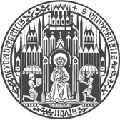## Statistical Mechanics, Analysis, and Probability

### Course at Universität Heidelberg Autumn 2013

#### Instructor

Manfred Salmhofer, Institut für Theoretische Physik

#### Contents

Most interesting models of Statistical Mechanics cannot be solved in closed form. Nevertheless, much useful, precise information can be gained using relatively elementary, but mathematically rigorous, methods. This course is to provide an introduction to these methods and results, as well as a perspective on mathematical structures that arise in that context.
Topics will include

• A bird's eye view of statistical mechanics
• Precise formulation of the main questions,
prototypical models
• DLR equations, mean-field theory
• The transfer matrix, the two-dimensional Ising model,
and the Perron-Frobenius theorem
• The Griffiths-Peierls argument
• Cluster expansions and analyticity
• Zeroes of partition functions, Yang-Lee theory,
the theory of Penrose and Lebowitz.
• O(N)-models, quantum spin systems.
• The Mermin-Wagner Theorem.
• The infrared bound and continuous symmetry breaking.
• Connections to large-deviation theory.

#### Prerequisites

Analysis and Linear Algebra. Basic ideas of classical and quantum mechanics. Previous exposure to ideas of statistical mechanics (e.g. in MKTP1) is helpful, but not necessary to follow this course.

#### Literature

will be announced in class

#### Coordinates

 Time: Tuesdays and Thursdays, 11:15-13:00 Place: Kleiner Hörsaal, Philosophenweg 12 starts October 15, 2013